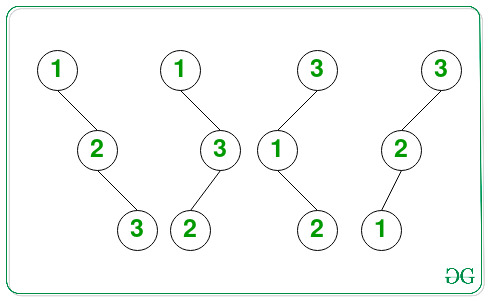Number of Binary Search Trees of height H consisting of H+1 nodes

• Last Updated : 19 May, 2021

Given a positive integer H, the task is to find the number of possible Binary Search Trees of height H consisting of the first (H + 1) natural numbers as the node values. Since the count can be very large, print it to modulo 109 + 7.

Examples:

Input: H = 2
Output: 4
Explanation: All possible BSTs of height 2 consisting of 3 nodes are as follows:Therefore, the total number of BSTs possible is 4.

Input: H = 6
Output: 64

Approach: The given problem can be solved based on the following observations:

• Only (H + 1) nodes are can be used to form a Binary Tree of height H.
• Except for the root node, every node has two possibilities, i.e. either to be the left child or to be the right child.
• Considering T(H) to be the number of BST of height H, where T(0) = 1 and T(H) = 2 * T(H – 1).
• Solving the above recurrence relation, the value of T(H) is 2H.

Therefore, from the above observations, print the value of 2H as the total number of BSTs of height H consisting of the first (H + 1) natural numbers.

Below is the implementation of the above approach:

C++

 // C++ program for the above approach   #include using namespace std;   const int mod = 1000000007;   // Function to calculate x^y // modulo 1000000007 in O(log y) int power(long long x, unsigned int y) {     // Stores the value of x^y     int res = 1;       // Update x if it exceeds mod     x = x % mod;       // If x is divisible by mod     if (x == 0)         return 0;       while (y > 0) {           // If y is odd, then         // multiply x with result         if (y & 1)             res = (res * x) % mod;           // Divide y by 2         y = y >> 1;           // Update the value of x         x = (x * x) % mod;     }       // Return the value of x^y     return res; }   // Function to count the number of // of BSTs of height H consisting // of (H + 1) nodes int CountBST(int H) {       return power(2, H); }   // Driver Code int main() {     int H = 2;     cout << CountBST(H);       return 0; }

Java

 // Java program for the above approach class GFG{       static int mod = 1000000007;   // Function to calculate x^y // modulo 1000000007 in O(log y) static long power(long x, int y) {           // Stores the value of x^y     long res = 1;       // Update x if it exceeds mod     x = x % mod;       // If x is divisible by mod     if (x == 0)         return 0;       while (y > 0)     {                   // If y is odd, then         // multiply x with result         if ((y & 1) == 1)             res = (res * x) % mod;           // Divide y by 2         y = y >> 1;           // Update the value of x         x = (x * x) % mod;     }       // Return the value of x^y     return res; }   // Function to count the number of // of BSTs of height H consisting // of (H + 1) nodes static long CountBST(int H) {     return power(2, H); }   // Driver code public static void main(String[] args) {     int H = 2;           System.out.print(CountBST(H)); } }   // This code is contributed by abhinavjain194

Python3

 # Python3 program for the above approach   # Function to calculate x^y # modulo 1000000007 in O(log y) def power(x, y):           mod = 1000000007           # Stores the value of x^y     res = 1       # Update x if it exceeds mod     x = x % mod       # If x is divisible by mod     if (x == 0):         return 0               while (y > 0):                   # If y is odd, then         # multiply x with result         if (y & 1):             res = (res * x) % mod           # Divide y by 2         y = y >> 1           # Update the value of x         x = (x * x) % mod           # Return the value of x^y     return res   # Function to count the number of # of BSTs of height H consisting # of (H + 1) nodes def CountBST(H):           return power(2, H)   # Driver Code H = 2   print(CountBST(H))   # This code is contributed by rohitsingh07052

C#

 // C# program for the above approach using System;   class GFG{       static int mod = 1000000007;   // Function to calculate x^y // modulo 1000000007 in O(log y) static long power(long x, int y) {           // Stores the value of x^y     long res = 1;       // Update x if it exceeds mod     x = x % mod;       // If x is divisible by mod     if (x == 0)         return 0;       while (y > 0)     {                   // If y is odd, then         // multiply x with result         if ((y & 1) == 1)             res = (res * x) % mod;           // Divide y by 2         y = y >> 1;           // Update the value of x         x = (x * x) % mod;     }       // Return the value of x^y     return res; }   // Function to count the number of // of BSTs of height H consisting // of (H + 1) nodes static long CountBST(int H) {           return power(2, H); }   // Driver code static void Main() {     int H = 2;           Console.Write(CountBST(H)); } }   // This code is contributed by abhinavjain194

Javascript



Output:

4

Time Complexity: O(log2H)
Auxiliary Space: O(1)

My Personal Notes arrow_drop_up
Recommended Articles
Page :